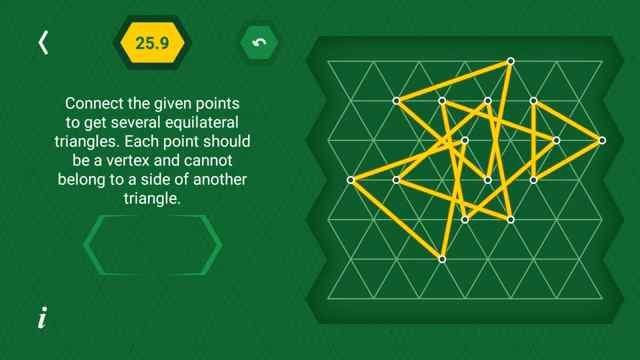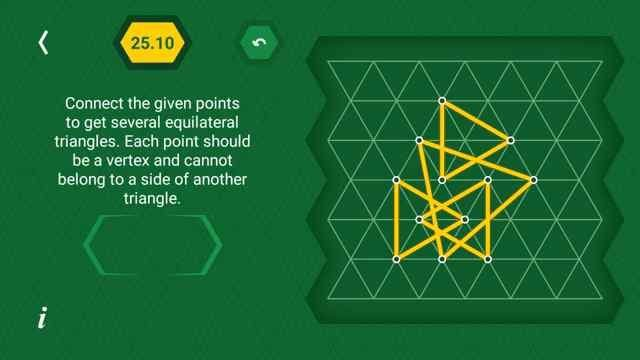# Pythagorea 60 degree Level 25.1 25.2 25.3 25.4 25.5 25.6 25.7 25.8 25.9 25.10 25.11 25.12 25.13 25.14 25.15 25.16

Pythagorea 60 janes triangle is an android/iOS app developed by Horis International Limited. This game is mostly focused on geometric puzzles and construction. The workspace is divided into triangular grids lines aligned 60° to each other, that’s why this game names as pythagorea 60°. You should know all the basic Math operations. All lines and shapes are drawn on a grid whose cells are equilateral triangles. Most of the game levels can be answered using natural intuition and bye some basic laws of geometry.

If you are here for levels other than ‘janes triangle’ Go to directory of all other levels at : http://www.puzzlegamemaster.com/pythagorea-60-complete/

Pythagorea 60 Jane’s Triangle 25.1 Solution:Pythagorea 60 Jane’s Triangle 25.2 Solution:Pythagorea 60 Jane’s Triangle 25.3 Solution:Pythagorea 60 Jane’s Triangle 25.4 Solution:Pythagorea 60 Jane’s Triangle 25.5 Solution:Pythagorea 60 Jane’s Triangle 25.6 Solution:Pythagorea 60 Jane’s Triangle 25.7 Solution:Pythagorea 60 Jane’s Triangle 25.8 Solution:Pythagorea 60 Jane’s Triangle 25.9 Solution:Pythagorea 60 Jane’s Triangle 25.10 Solution:Pythagorea 60 Jane’s Triangle 25.11 Solution:Pythagorea 60 Jane’s Triangle 25.12 Solution:Pythagorea 60 Jane’s Triangle 25.13 Solution:Pythagorea 60 Jane’s Triangle 25.14 Solution:Pythagorea 60 Jane’s Triangle 25.15 Solution:Pythagorea 60 Jane’s Triangle 25.16 Solution: